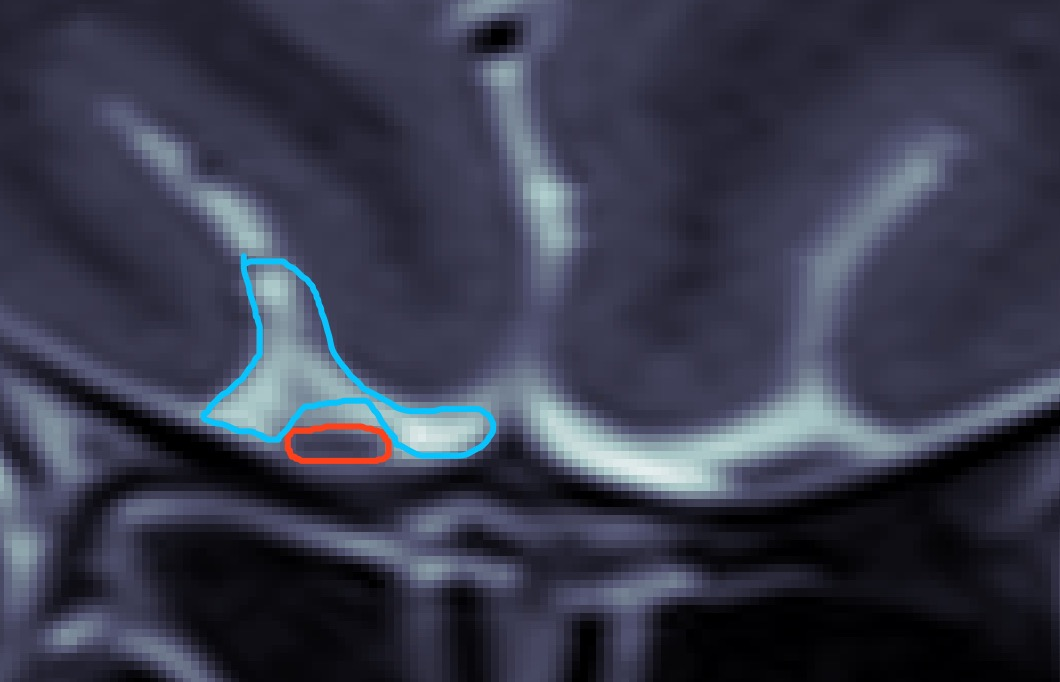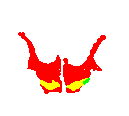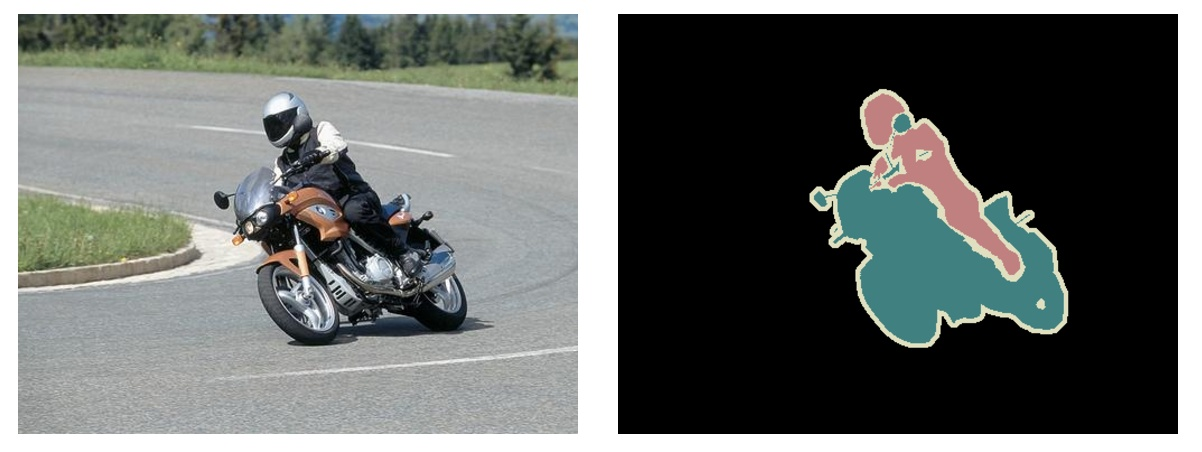# 优雅的代价 - 记一次多分类分割的实现epi_img = nib.load(nii_path)
epi_img_data = []
epi_img_data.append(epi_img.get_fdata())  # 嗅球label
epi_img_data.append(white_epi_img.get_fdata())  # 白色区域label
_,_,frames = epi_img_data.shape #eg:a:896,b:896,frames:19(代表病人一有19张图)
for frame in range(frames):
label2write = np.zeros((896, 896, 3), np.uint8)
label_path = os.path.join(out_folder, names[frame] + ".png")
for i in range(2):
label = epi_img_data[i][:,:,frame]
label = label*255
label = np.rot90(label, k=1, axes=(1,0))  #图像旋转
label = np.fliplr(label)                  #图像翻转
if not label.shape == 896:
label=cv2.resize(label,(896,896))
label2write[:,:,i+1] = label  # 默认BGR通道，嗅球存G通道，白色区域存R通道
cv_imwrite(label_path, label2write)   #保存整个完整嗅球相关组织人为标记标签的图片